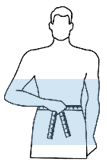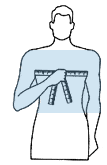#### GUIDE FOR GENRE MEASURE, STANDARDIZATION OF CLOTHING AND UNIFORM MEASURES:

MALE MEASURES: How to find measures for male mannequins:
How to measure Pants, Shorts and Shorts?Put the measuring tape stretched around the waist.Check the number and divide by 2..

Example: If the value is 84, then divide by two and find the value: 42 (84/2).

We have sizes from 36 to 60.
How to measure polos, tshirts, shirts, coats, vests, jackets?Put measuring tape, streched under the armpits, around your chest.Get the value and divide by 2.

Example: If the value found on the tape is 110, then divide by 2 and find the measurement: 55 (110/2).

 Sizes: XS S M L XL XXL XXXL Width (A) 46 49 52 56 59 62 65 Height (B) 67 69 71 73 75 77 79

#### GUIDE FOR GENRE MEASURE, STANDARDIZATION OF CLOTHING AND UNIFORM MEASURES:

MALE MEASURES: How to find measures for male mannequins:
How to measure Pants, Shorts and Shorts?Put the measuring tape stretched around the waist.Check the number and divide by 2..

Example: If the value is 84, then divide by two and find the value: 42 (84/2).

We have sizes from 36 to 60.
How to measure polos, tshirts, shirts, coats, vests, jackets?Put measuring tape, streched under the armpits, around your chest.Get the value and divide by 2.

Example: If the value found on the tape is 110, then divide by 2 and find the measurement: 55 (110/2).

 Sizes: XS S M L XL XXL XXXL Width (A) 46 49 52 56 59 62 65 Height (B) 67 69 71 73 75 77 79

#### GUIDE FOR GENRE MEASURES, STANDARDIZATION OF CLOTHES AND UNIFORMS:

FEMALE MEASURES: How to find measures for female mannequins:
How to measure Pants, Shorts and Skirts?The tape measure is placed around the most protruding part of the hip.
- Sum to the result + 4.
- Divide the total by 2
- And finally subtract 10 cm

Example: If the value of the tape measure was 96, add + 4 (96 + 4 = 100).
Divide this result now by 2 (100/2 = 50) and always substrate 10.
The final result would be, 40.

We have sizes from 36 to 60.
How to measure Polos, Blouses, Jackets and Dresses?Place the measuring tape stretched below the armpits around the bust.
- Add to this number + 10 cm
- This total is divided by 2
- Subtract 8 cm from the result.

Example: If the value found on the tape measure was 100, then we divide it by 2 and find the measure: 50 (100/2). Subtract 8 cm from the result. the number found would be 42.

 Sizes: XS S M L XL XXL XXXL Width (A) 40,5 43 46 48,5 51,5 55 65 Height (B) 59 60 61 62 63 64 65

#### GUIDE FOR GENRE MEASURE, STANDARDIZATION OF CLOTHING AND UNIFORM MEASURES:

MALE MEASURES: How to find measures for male mannequins:
How to measure Pants, Shorts and Shorts?Put the measuring tape stretched around the waist.Check the number and divide by 2..

Example: If the value is 84, then divide by two and find the value: 42 (84/2).

We have sizes from 36 to 60.
How to measure polos, tshirts, shirts, coats, vests, jackets?Put measuring tape, streched under the armpits, around your chest.Get the value and divide by 2.

Example: If the value found on the tape is 110, then divide by 2 and find the measurement: 55 (110/2).

 Sizes: XS S M L XL XXL XXXL Width (A) 46 49 52 56 59 62 65 Height (B) 67 69 71 73 75 77 79

#### GUIDE FOR GENRE MEASURES, STANDARDIZATION OF CLOTHES AND UNIFORMS:

FEMALE MEASURES: How to find measures for female mannequins:
How to measure Pants, Shorts and Skirts?The tape measure is placed around the most protruding part of the hip.
- Sum to the result + 4.
- Divide the total by 2
- And finally subtract 10 cm

Example: If the value of the tape measure was 96, add + 4 (96 + 4 = 100).
Divide this result now by 2 (100/2 = 50) and always substrate 10.
The final result would be, 40.

We have sizes from 36 to 60.
How to measure Polos, Blouses, Jackets and Dresses?Place the measuring tape stretched below the armpits around the bust.
- Add to this number + 10 cm
- This total is divided by 2
- Subtract 8 cm from the result.

Example: If the value found on the tape measure was 100, then we divide it by 2 and find the measure: 50 (100/2). Subtract 8 cm from the result. the number found would be 42.

 Sizes: XS S M L XL XXL XXXL Width (A) 40,5 43 46 48,5 51,5 55 65 Height (B) 59 60 61 62 63 64 65

#### GUIDE FOR GENRE MEASURES, STANDARDIZATION OF CLOTHES AND UNIFORMS:

FEMALE MEASURES: How to find measures for female mannequins:
How to measure Pants, Shorts and Skirts?The tape measure is placed around the most protruding part of the hip.
- Sum to the result + 4.
- Divide the total by 2
- And finally subtract 10 cm

Example: If the value of the tape measure was 96, add + 4 (96 + 4 = 100).
Divide this result now by 2 (100/2 = 50) and always substrate 10.
The final result would be, 40.

We have sizes from 36 to 60.
How to measure Polos, Blouses, Jackets and Dresses?Place the measuring tape stretched below the armpits around the bust.
- Add to this number + 10 cm
- This total is divided by 2
- Subtract 8 cm from the result.

Example: If the value found on the tape measure was 100, then we divide it by 2 and find the measure: 50 (100/2). Subtract 8 cm from the result. the number found would be 42.

 Sizes: XS S M L XL XXL XXXL Width (A) 40,5 43 46 48,5 51,5 55 65 Height (B) 59 60 61 62 63 64 65

#### GUIDE FOR GENRE MEASURE, STANDARDIZATION OF CLOTHING AND UNIFORM MEASURES:

MALE MEASURES: How to find measures for male mannequins:
How to measure Pants, Shorts and Shorts?Put the measuring tape stretched around the waist.Check the number and divide by 2..

Example: If the value is 84, then divide by two and find the value: 42 (84/2).

We have sizes from 36 to 60.
How to measure polos, tshirts, shirts, coats, vests, jackets?Put measuring tape, streched under the armpits, around your chest.Get the value and divide by 2.

Example: If the value found on the tape is 110, then divide by 2 and find the measurement: 55 (110/2).

 Sizes: XS S M L XL XXL XXXL Width (A) 46 49 52 56 59 62 65 Height (B) 67 69 71 73 75 77 79

#### GUIDE FOR GENRE MEASURES, STANDARDIZATION OF CLOTHES AND UNIFORMS:

FEMALE MEASURES: How to find measures for female mannequins:
How to measure Pants, Shorts and Skirts?The tape measure is placed around the most protruding part of the hip.
- Sum to the result + 4.
- Divide the total by 2
- And finally subtract 10 cm

Example: If the value of the tape measure was 96, add + 4 (96 + 4 = 100).
Divide this result now by 2 (100/2 = 50) and always substrate 10.
The final result would be, 40.

We have sizes from 36 to 60.
How to measure Polos, Blouses, Jackets and Dresses?Place the measuring tape stretched below the armpits around the bust.
- Add to this number + 10 cm
- This total is divided by 2
- Subtract 8 cm from the result.

Example: If the value found on the tape measure was 100, then we divide it by 2 and find the measure: 50 (100/2). Subtract 8 cm from the result. the number found would be 42.

 Sizes: XS S M L XL XXL XXXL Width (A) 40,5 43 46 48,5 51,5 55 65 Height (B) 59 60 61 62 63 64 65

#### GUIDE FOR GENRE MEASURE, STANDARDIZATION OF CLOTHING AND UNIFORM MEASURES:

MALE MEASURES: How to find measures for male mannequins:
How to measure Pants, Shorts and Shorts?Put the measuring tape stretched around the waist.Check the number and divide by 2..

Example: If the value is 84, then divide by two and find the value: 42 (84/2).

We have sizes from 36 to 60.
How to measure polos, tshirts, shirts, coats, vests, jackets?Put measuring tape, streched under the armpits, around your chest.Get the value and divide by 2.

Example: If the value found on the tape is 110, then divide by 2 and find the measurement: 55 (110/2).

 Sizes: XS S M L XL XXL XXXL Width (A) 46 49 52 56 59 62 65 Height (B) 67 69 71 73 75 77 79